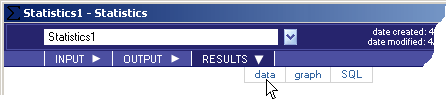# Statistics - RESULTS - Data

## Teradata Warehouse Miner User Guide - Volume 1Introduction and Profiling

brand
Software
prodname
vrm_release
5.4.4
category
User Guide
featnum
B035-2300-077K
1. On the Statistics dialog box, click on RESULTS.
2. Click on data (note that the RESULTS tab will be grayed-out/disabled until after the analysis is completed).
Statistics > Results > DataResults data, if any, is displayed in a data grid as described in RESULTS Tab.

The following is a description of the results returned by the analysis, depending on the options selected. If an output table is created, the columns in bold below comprise the Unique Primary Index (UPI) of the output table.

Statistics Result Data
Name Type Definition
COL Group By Column Type Column(s) will be created only if the Group By option is specified. Multiple columns, if specified, will be created or displayed as the column name. These column(s) will contain the unique values that the group by column takes on.
xtbl VARCHAR (30) Table that the variable for the statistics operation resides in, as specified by the “table” parameter.
xcol VARCHAR (30) Variable that the statistics operation will be run against, as specified by the “column” parameter.
xcnt FLOAT The total number of occurrences of this variable.
xmin FLOAT The minimum value of the variable. Created only if the Minimum Value option is selected.
xmax FLOAT The maximum value of the variable. Created only if the Maximum Value option is selected.
xmean FLOAT The arithmetic mean of the variable. Created only if the Mean Value option is selected.
xstd FLOAT The standard deviation of the variable. Created only if the Standard Deviation option is selected.
xskew FLOAT The skewness of the variable. Created only if the Skewness option is selected.
xkurt FLOAT The kurtosis of the variable. Created only if the Kurtosis option is selected.
xste FLOAT The standard error of the variable. Created only if the Standard Error option is selected.
xcv FLOAT The coefficient of variance of the variable. Created only if the Coefficient of Variance option is selected.
xvar FLOAT The variance of the variable. Created only if the Variance option is selected.
xsum FLOAT The sum of the variable. Created only if the Sum option is selected.
xuss FLOAT The uncorrected sums of squares of the variable. Created only if the Uncorrected Sums of squares option is selected.
xcss FLOAT The corrected sums of squares of the variable. Created only if the Corrected Sums of squares option is selected.
xnull FLOAT Total number of rows where this variable takes on a null value. Created only if the Values option is selected.
xunique FLOAT Total number of rows where this variable takes on a unique value. Created only if the Values option is selected.
xblank FLOAT Total number of rows where this variable is blank. Created only if the Values option is selected.
xzero FLOAT Total number of rows where this variable is equal to 0. Created only if the Values option is selected.
xpos FLOAT Total number of rows where this variable has a positive value. Created only if the Values option is selected.
xneg FLOAT Total number of rows where this variable has a negative value. Created only if the Values option is selected.
xmode FLOAT Modal, or most frequently occurring value that the variable is equal to. Note that this is the minimum modal value; if there are multiple modes, xnbrmodes will exist and contain the total number of modal values. Created only if the Modes option is selected.
xmode_cnt FLOAT The number of times the modal value occurs. If the group option is used, the count specified is within each group. Created only if the Modes option is selected.
xmode_pct FLOAT The percentage that the modal value occurs with respect to all selected records. If the group option is used, the percentage within each group. Created only if the Modes option is selected.
xnbrmodes FLOAT The total number of modal values. Created only if the Modes option is selected.
xpctileN FLOAT Percentiles, deciles, quartiles and tertiles of the variable. N is defined as follows:
• Bottom 10 percentiles (N=0-9)
• Deciles (N=10, 20, 30, 40, 50, 60, 70, 80, 90)
• Quartiles (N=25, 50, 75)
• Tertiles (N=33,67)
• Top 10 percentiles (N=91-100)

Created only if the Quantiles option is selected.

xmin_N FLOAT The five smallest occurring values that the variable takes on. Created only if the Rank option is selected.

N=1-5

xmincnt_N FLOAT The number of times that the variable takes on the five smallest occurring values. Created only if the Rank option is selected.

N=1-5

xmax_N FLOAT The five largest occurring values that the variable takes. Created only if the Rank option is selected.

N=1-5

xmaxcnt_N FLOAT The number of times that the variable takes on the five largest occurring values. Created only if the Rank option is selected.

N=1-5